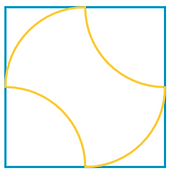Squares

Rotating Square

The dot represents the center of the square. Find the position of the blue square such that the overlapping area (as a fraction of the orange square's area) is maximum.Circle, Square, Circle

The blue quadrilateral is a square. What is the ratio of the two circles' areas?Four Arcs and a Square

All arcs are congruent. What is the area contained by the four arcs as a fraction of the square's area?Circles vs. Squares

The two large circles have radii of 1. The dodecagon is made up of five squares. Which design contains the largest area: the four circles or the dodecagon?3 Vertices, 2 Midpoints

The blue segments connect a vertex to the midpoint of a side. What fraction of the square's area is yellow?4 Vertices, 4 Midpoints

The blue segments connect a vertex to the midpoint of a side. What fraction of the square's area is yellow?